• ## matlab画2d曲线命令

千次阅读 2012-03-13 18:59:42
plot  - 2-D line plot ... a real number.... the same axes.... a matrix and the other ...In the MATLAB workspace browser, select one or more variables. Choose the plot type from the   menu.

plot -2-D
line plot

Syntax

plot(Y)
plot(X1,Y1,...,Xn,Yn)
plot(X1,Y1,LineSpec,...,Xn,Yn,LineSpec)
plot(...,'PropertyName',PropertyValue,...)
plot(axes_handle,...)
h = plot(...)

Description

plot(Y) plots
the columns of Y versus the index of each value when Y is
a real number. For complex Y, plot(Y) is
equivalent toplot(real(Y),imag(Y)).

plot(X1,Y1,...,Xn,Yn) plots
each vector Yn versus vector Xn on
the same axes. If one of Yn or Xn is
a matrix and the other is a vector, it plots the vector versus the matrix row or column with a matching dimension to the vector. If Xn is
a scalar and Yn is a vector, it plots discrete Yn points
vertically at Xn. If Xn or Yn are
complex, imaginary components are ignored. If Xn or Yn are
matrices, they must be 2-D and the same size, and the columns of Yn are plotted against the columns of Xn. plot automatically
chooses colors and line styles in the order specified by ColorOrder and LineStyleOrder properties
of current axes.

plot(X1,Y1,LineSpec,...,Xn,Yn,LineSpec) plots
lines defined by the Xn,Yn,LineSpec triplets, where LineSpec specifies
the line type, marker symbol, and color. You can mix Xn,Yn,LineSpec triplets with Xn,Yn pairs: plot(X1,Y1,X2,Y2,LineSpec,X3,Y3).

plot(...,'PropertyName',PropertyValue,...) manipulates
plot characteristics by setting lineseries
properties (of lineseries graphics objects created by plot).
Enter properties as one or more name-value pairs. Property name-value pairs apply to all the lines plotted. You cannot specify name-value pairs for each set of data.

plot(axes_handle,...) plots
using axes with the handle axes_handle instead of the current axes (gca).

h
= plot(...) returns a column vector of handles to lineseries objects, one handle per line.

Tips

Plot data can include NaN and inf values,
which cause breaks in the lines drawn. For example,
plot([1:5,NaN,6:10])

Skips the sixth element and resumes line drawing at the seventh element with the Y value of 6.

Examples

Plot a sine curve.
x = -pi:.1:pi;
y = sin(x);
plot(x,y)Create line plot using specific line width, marker color, and marker size.
x = -pi:pi/10:pi;
y = tan(sin(x)) - sin(tan(x));
plot(x,y,'--rs','LineWidth',2,...
'MarkerEdgeColor','k',...
'MarkerFaceColor','g',...
'MarkerSize',10)Modify axis tick marks and tick labels and annotate the graph.
x = -pi:.1:pi;
y = sin(x);
plot(x,y)
set(gca,'XTick',-pi:pi/2:pi)
set(gca,'XTickLabel',{'-pi','-pi/2','0','pi/2','pi'})
title('Sine Function');
xlabel('Radians');
ylabel('Function Value');Add a plot title, axis labels, and annotations.
x = -pi:.1:pi;
y = sin(x);
p = plot(x,y)
set(gca,'XTick',-pi:pi/2:pi)
set(gca,'XTickLabel',{'-pi','-pi/2','0','pi/2','pi'})
xlabel('-\pi \leq \Theta \leq \pi')
ylabel('sin(\Theta)')
title('Plot of sin(\Theta)')
% \Theta appears as a Greek symbol (see String)
% Annotate the point (-pi/4, sin(-pi/4))
text(-pi/4,sin(-pi/4),'\leftarrow sin(-\pi\div4)',...
'HorizontalAlignment','left')
% Change the line color to red and
% set the line width to 2 points
set(p,'Color','red','LineWidth',2)Plot multiple line plots on the same axes.
plot(sin(x));
% hold axes and all lineseries properties, such as
% ColorOrder and LineStyleOrder, for the next plot
hold all
plot(sin(x+(pi/4)));Set line color to be always black and line style order to cycle through solid, dash-dot, dash-dash, and dotted line styles.
set(0,'DefaultAxesColorOrder',[0 0 0],...
'DefaultAxesLineStyleOrder','-|-.|--|:')
plot(sin(x))
hold all
plot(cos(x))
hold all
plot(log(abs(x)))Alternatives

To plot variables in the MATLAB workspace:

In the MATLAB workspace browser, select one or more variables.

Choose the plot type from themenu.

展开全文plot properties vector matrix
• 实验名称用MATLAB进行系统频率特性曲线绘制实验时间2014年12月10日学生姓名实验地点070312同组人员无专业班级新能源1201B1、实验目的1)熟练掌握使用MATLAB命令绘制控制系统奈氏图的方法；2)熟练掌握使用MATLAB命令...
实验名称用MATLAB进行系统频率特性曲线绘制实验时间2014年12月10日学生姓名实验地点070312同组人员无专业班级新能源1201B1、实验目的1)熟练掌握使用MATLAB命令绘制控制系统奈氏图的方法；2)熟练掌握使用MATLAB命令绘制控制系统伯德图的方法；3)加深理解控制系统奈氏稳定判据的实际应用。2、实验主要仪器设备和材料：计算机一台matlab软件2010a版本3、实验内容和原理：原理：(1)幅相频率特性曲线，以角频率w为参变量，当w从0变化时，频率特性构成的向量在复平面上描绘的曲线称为幅相频率特性曲线。(2)奈氏判据，奈氏判据是利用系统开环频率特性来判断闭环系统稳定性的一个判据，便于研究当系统结构改变时对系统稳定性的影响。其内容是：反馈控制系统稳定的充分必要条件是当w从-变到+时，开环系统的奈氏判据GH不穿过()10j-，点逆时针包围临界点()10j-，的圈数R等于开环传递函数的正实部极点数P。(1)对于开环稳定的系统，闭环系统稳定的充分必要条件是：开环系统的奈氏曲线GH不包围()10j-，点。反之，则闭环系统是不稳定的。(2)对于开环不稳定的系统，有p个开环极点位于右半s平面，则闭环系统稳定的充分必要条件是：当w从-变到+时，开环系统的奈氏判据GH逆时针包围()10j-，点p次。内容：1)绘制控制系统奈氏图格式一：nyquist(num,den)格式二:nyquist(num,den,w)格式三：[re,im,w]=nyquist(num,den)a.开环开环传递函数()210210Gsss=++，绘制其Nyquist图。b.已知()()320.520.5GsHssss=+++，绘制Nyquist图，判定系统的稳定性。c.已知系统开环传递函数为()12(1)(1)kTGssTs+=+，要求：分别作出12TT>和12TT<时的Nyquist图。
展开全文• [MATLAB]使用命令文件，出下列分段函数所表示的曲线。 y=x+1 (x<0) y=1 (0<=x<1) y=x^3 (1<=x) x=-1:0.01:2; y=x+1.*(x<0)+1*(0<=x&x<1)+x.^3.*(1<=x); plot(x,y,'r'); grid on title...
[MATLAB]使用命令文件，画出下列分段函数所表示的曲线。
y=x+1 (x<0)
y=1  (0<=x<1)
y=x^3   (1<=x)
x=-1:0.01:2;
y=x+1.*(x<0)+1*(0<=x&x<1)+x.^3.*(1<=x);
plot(x,y,'r');
grid on
title('分段函数所表示的曲线')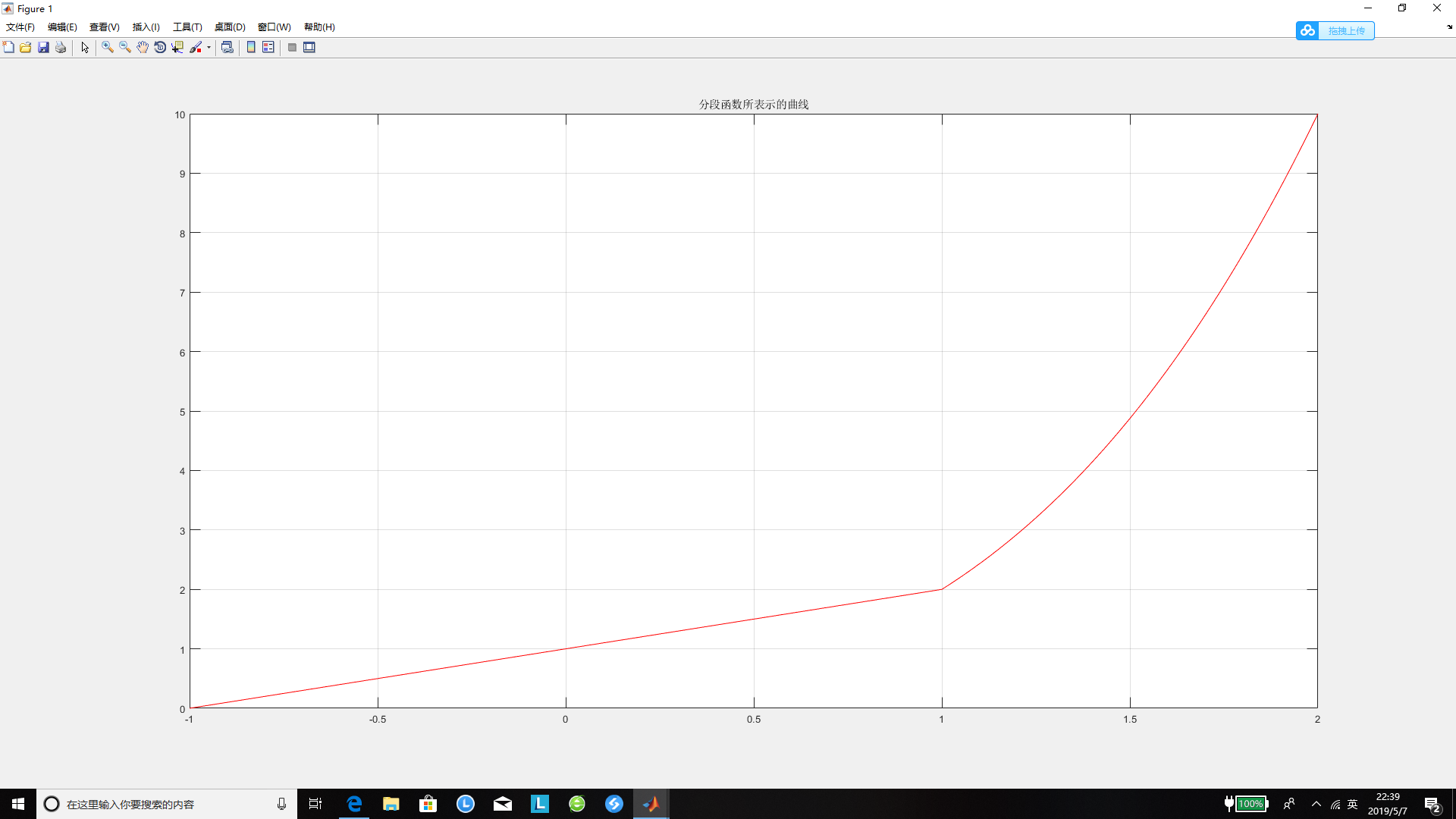展开全文• matlab 调用plot3函数来绘制三维曲线图，调用格式如下： plot3(x1,y1,z1,’PropertyName’,’PropertyValue’...); PropertyName表示属性名，可以是颜色，线型，线宽等属性，PropertyValue表示属性值； 先给自变量...
matlab 调用plot3函数来绘制三维曲线图，调用格式如下：
plot3(x1,y1,z1,’PropertyName’,’PropertyValue’...);
PropertyName表示属性名，可以是颜色，线型，线宽等属性，PropertyValue表示属性值；

先给自变量t赋值；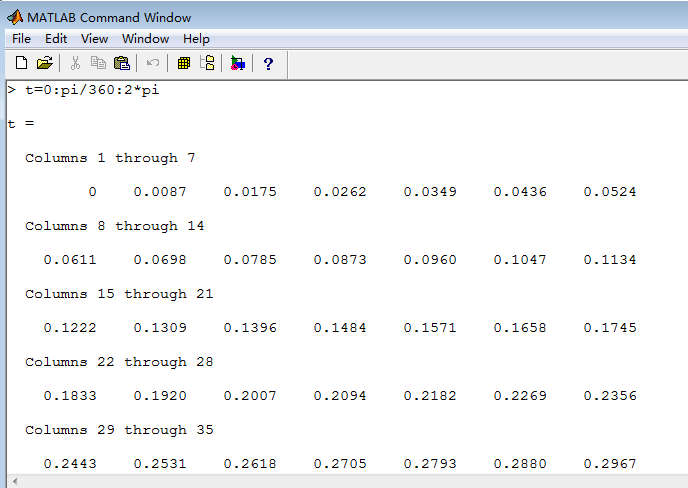定义一个函数x；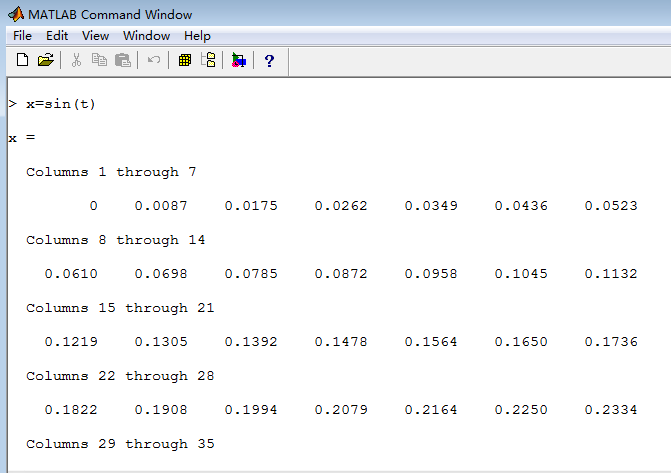定义一个函数y；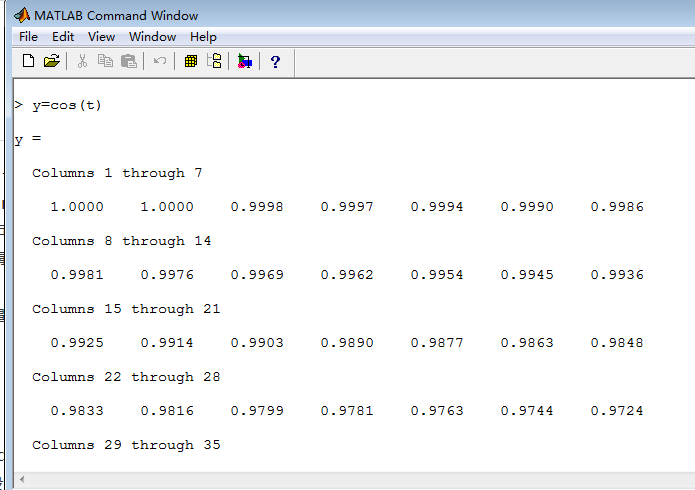定义函数z，是前面2个函数的函数；这样z有2个自变量，是一个三维的值；

清屏的话使用clc命令；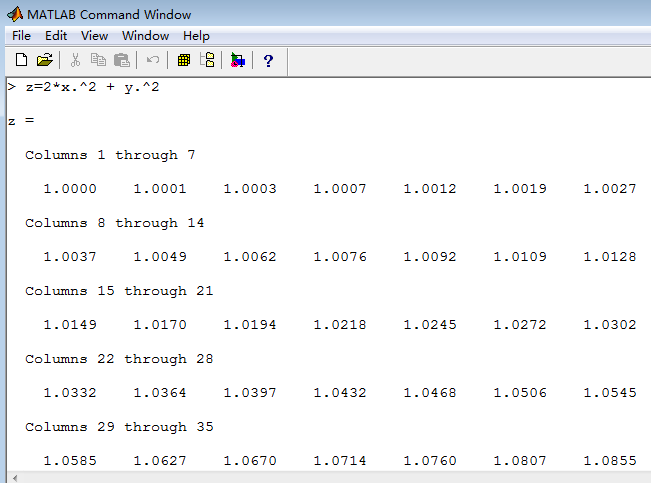然后画一下z；plot3(x,y,z,'Color','r','LineWidth',3)；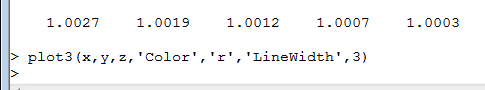一个红色，三像素宽的三维曲线出来了；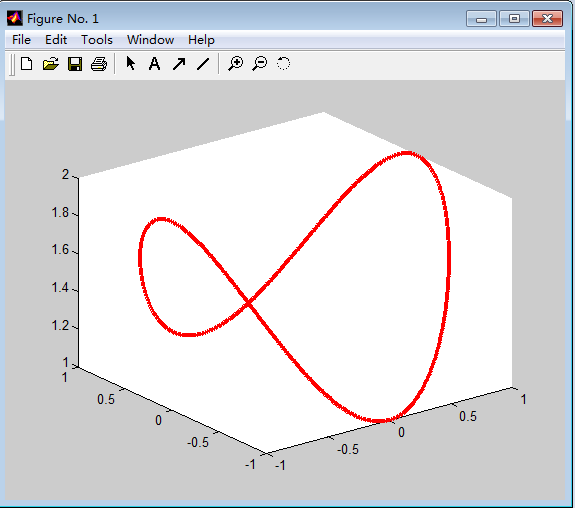展开全文plot
• MATLAB画函数曲线 2013年8月11日 命令funtool 这是单变量函数分析的交互界面，比较方便，特别适用于y=f（x）型，即y与x分开的函数形式。见下图 转载于:...
• MATLAB画双纵坐标具有两个纵坐标标度的图形在MATLAB中，如果需要绘制出具有不同纵坐标标度的两个图形，可以使用plotyy绘图函数。调用格式为：plotyy(x1,y1,x2,y2)其中x1,y1对应一条曲线，x2,y2对应另一条曲线。横...
• MATLAB画双纵坐标plotyy的用法对数坐标MATLAB画双纵坐标具有两个纵坐标标度的图形在MATLAB中，如果需要绘制出具有不同纵坐标标度的两个图形，可以使用plotyy绘图函数。调用格式为：plotyy(x1,y1,x2,y2)其中x1,y1对应...
• ## MATLAB 常用绘图命令

千次阅读 2019-08-14 10:46:03
引言 MATLAB提供了强大的图形用户界面，在许多应用中，常常要用绘图功能来实现数据的显示和分析。在控制系统仿真中，也常常用到绘图,如绘制系统的响应...同理，输入“ help graph3d”可得到所有三维图形的命令和帮...
• 1.在plot曲线时，有时想在一张图上重合多条曲线，我们只需要在画图命令之前加上hold on就好，比如： t = 1:0.1:10； y1 = sin(2*pi*t); y1 = cos(2*pi*t); plot(y1); hold on; plot(y2); 运行结果如下： ...plot figure hold on subplot
• 具有两个纵坐标标度的图形在MATLAB中，如果需要绘制出具有不同纵坐标标度的两个图形，可以使用plotyy绘图函数。调用格式为：plotyy(x1,y1,x2,y2)其中x1,y1对应一条曲线，x2,y2对应另一条曲线。横坐标的标度相同，纵...
• 输入fvtool命令根据滤波器系数出滤波器特性曲线，f = w*fs/2 (f为实际频率，w为横坐标) 滤波器系数成比例后，滤波后增益也成比例增大 比如，我用fvtool出滤波器特性曲线后是这个效果（我设置的fs为4.8k，则通...
• 话题：如何利用matlab的plotyy函数双坐标图??回答：????具有两个纵坐标标度的图形 在MATLAB中,如果需要绘制出具有不同纵坐标标度的两个图形,可以使用plotyy绘图函数。调用格式为: plotyy(x1,y1,x2,y2) 其中x1,y1...
• MATLAB符号绘图——相见恨晚的MATLAB绘图...因为着些函数都是基于已有的数据绘制图形的，有些时候我们只知道某个函数的表达式，而且这个表达式比较复杂，而项目要求出这个图形的曲线，怎么办呢？没关系，MATLAB是个数据可视化
• #matlab —— cftool曲线拟合# 1.输入数据： 2.启动cftool工具箱：输入命令cftool 3.导入数据 功能简介 第一个是“main plot”，出主要的图 第二个是“Residuals plot”，出残差图 第三个是“Contour plot”，...
• 有那些相见恨晚的MATLAB绘图命令MATLAB的绘图功能可谓非常的强大，常用的有plot,plot3,subplot,scatter等等，这些函数有许多的参数设置，可以出各种需求的图。但是这些强大的绘图命令也有无能为力的时候。为什么呢...
• ## Matlab画三维图笔记

万次阅读 多人点赞 2015-06-09 23:02:10
plot3 基本的三维曲线图绘制...plot3(X,Y,Z)，X,Y,Z均为相同大小的矩阵,均为m*n的矩阵时，plot3命令将绘得m条曲线，每条曲线均为X，Y，Z列向量为（x,y,z）坐标值的曲线 例 三维圆柱形螺旋线,x,y,z均为相同长度的向量 clplot
• 接上文：Matlab精彩画图示例：多子图Matlab精彩画图示例：图上图对比查看不同曲线，除了采用图上图、多子图的方式，还可以采用将多条曲线画到一张图里查看，这样更方便、直观，尤其对于曲线族的绘制。这里有两种方式...
• 有那些相见恨晚的MATLAB绘图命令MATLAB的绘图功能可谓非常的强大，常用的有plot,plot3,subplot,scatter等等，这些函数有许多的参数设置，可以出各种需求的图。但是这些强大的绘图命令也有无能为力的时候。为什么呢...
• 教你如何使用matlab，非常全面的。 目录 译者序 前言 第1章 MATLAB是什么 1 1.1 MATLAB能做什么 1 1.2 MATLAB实例 1 1.3 MATLAB帮助 10 第2章 MATLAB启动 11 2.1 启动和退出MATLAB 11 2.2 MATLAB中矩阵和多维矩阵...
• 函数polt是针对向量或矩阵的列来绘制曲线的，其命令格式： （1）plot（x）当x是一向量时，以其元素为纵坐标，其序号为横坐标。 （2）plot（x，y） （3）plot（x，y1，x，y2，...）绘制多条曲线 A=[ 2551.35 ,...
• matlab是一款功能强大的通用工程数学软件。利用matlab的作图功能可以轻而易举地绘制出各种复杂函数图形。利用ezplot甚至可以直接...那么matlab究竟有没有用来绘制诸如F(x,y,z)=0的命令呢？答案毫无疑问是肯定的。事实上函数
• MATLAB plot用指定的8种字符可以使得曲线呈现8种不同的颜色，这8种系统颜色分别是 颜色 字符 红 r 绿 g 蓝 b 青绿 c 洋红 m 黄 y 黑 k 白 w 例如命令 plot(x, y, '...plot
• 接上文：Matlab精彩画图示例：多子图Matlab精彩画图示例：图上图对比查看不同曲线，除了采用图上图、多子图的方式，还可以采用将多条曲线画到一张图里查看，这样更方便、直观，尤其对于曲线族的绘制。这里有两种方式...
• 下面将介绍MATLAB基本xy平面及xyz空间的各项绘图命令，包含一维曲线及二维曲面的绘制、列印及存档。 plot 是绘制一维曲线的基本函数，但在使用此函数之前，我们需先定义曲线上每一点的x及y座标。 下例可出一条...
• 一基本绘图函数1....如果Y是矩阵，则出Y中各列相对于行号的曲线.plot(X,Y), 如X，Y为同维实向量，则绘出横坐标为X纵坐标为Y的曲线 plot(X,Y, 'Linespec'), 按Linespec所规定的线型、标记点、颜色...
• 在绘图命令中应该如何确定横坐标和纵坐标的标度尺寸（自己定范围） 如何在一个坐标中绘制多条曲线，并用不同的颜色区别。 希望得到答案，谢谢！！！！！！！！！！！&nbsp;help plot help axis&nbsp;axis ...
• matplotlib 是python最著名的绘图库，它提供了一整套和matlab相似的命令API，十分适合交互式地行制图。而且也可以方便地将它作为绘图控件，嵌入GUI应用程序中。它的文档相当完备，并且Gallery页面中有上百幅缩略图，...
• 在绘图命令中应该如何确定横坐标和纵坐标的标度尺寸（自己定范围） 如何在一个坐标中绘制多条曲线，并用不同的颜色区别。 希望得到答案，谢谢！！！！！！！！！！！  help plot help axis  axis ('xlabel','......

# matlab画曲线命令matlab 订阅